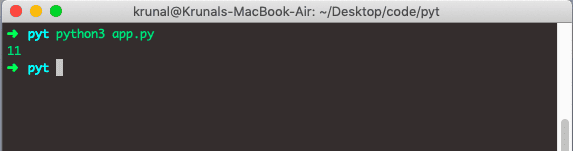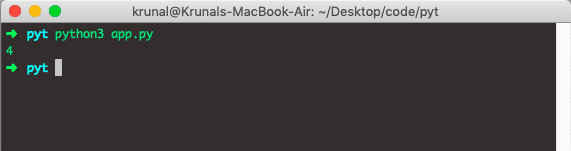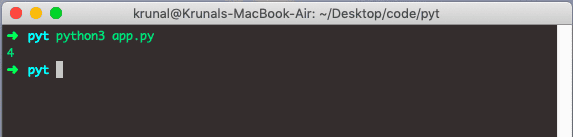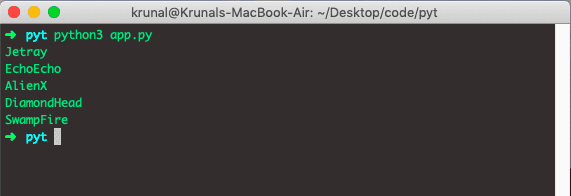# Python len Function: The Complete Guide

The len() function on any iterable objects. It returns an integer which is the length of that iterable object.

## Python length

Python len() is a built-in function that returns the length of any iterable objects. It returns the no. of characters of String; it returns the total number of elements in a list or dictionary.

### Syntax

`len(any iterable object)`

The only required argument is an iterable object which can be a list, String, or dictionary.

## Python string length

To find a string length in Python, use the len() function. We can calculate the length of the String using the following code.

```# app.py

strA = 'AppDividend'
print(len(strA))```

See the output.The output is 11, which means that String AppDividend contains 11 characters.

## Python list length

To find a list length in Python, use the len() function. We can calculate the length of the List. Calculating the length of the List means that we need to count the number of elements the List has.

See the following code.

```# app.py

listA = ['PlayStation', 'Xbox', 'PSP', 'Wii']
print(len(listA))```

See the output.## Python dictionary length

To find a dictionary length in Python, use the len() function. Calculating the dictionary’s length means we need to count the number of items the dictionary has.

See the below code.

```# app.py

dictA = {
'name': 'Krunal Lathiya',
'university': 'GTU',
'enrollmentno': 110470116021,
'college': 'VVP Engineering College'
}
print(len(dictA))```

See the below output.## Use len() function in For loop

We can use the len() function in For loop. Let’s see the below example.

```# app.py

ben10 = ['Jetray', 'EchoEcho', 'AlienX','DiamondHead', 'SwampFire']
i = 0
for i in range(0, len(ben10)):
print(ben10[i])```

In the above code, we have defined one List and then iterate that List from 0 to the length of the List. Then we printed the aliens one by one.

See the below output.That’s it for this tutorial.

### 1 thought on “Python len Function: The Complete Guide”

1.Thanks for the post